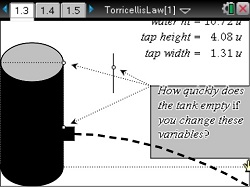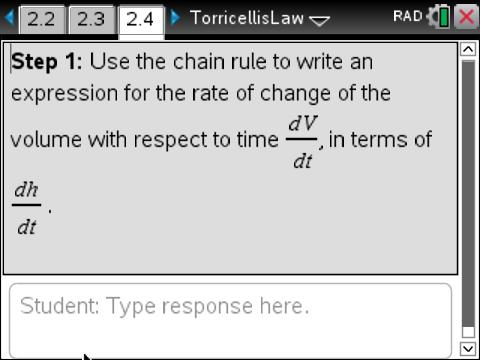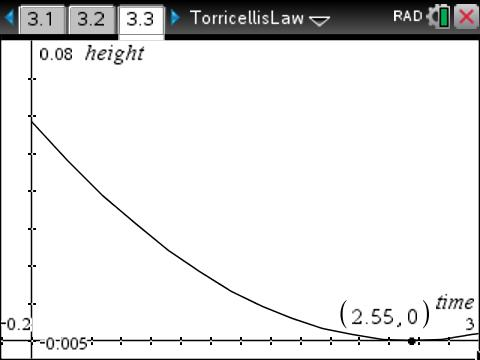# Activities

••• ##### Subject Area

• Math: Calculus: Differential Equations

• ##### Author9-12

45 Minutes

• ##### Device
•TI-Nspire™ CX/CX II
•TI-Nspire™ CX CAS/CX II CAS
• TI-Nspire™ Navigator™
• TI-Nspire™
• TI-Nspire™ CAS
• ##### Software

TI-Nspire™
TI-Nspire™ CAS

3.0

• ##### Other Materials
By Stephen Arnold

## Torricelli's Law

#### Activity Overview

Students examine the velocity of water flowing through the tap of a tank, finding an equation to model the height of the water in the tank as the tank is drained.

#### Key Steps

•Students investigate Torricelli’s Law. This law describes the relationship between the velocity of fluid leaving a container under the force of gravity and the height of the fluid.

Students explore this relationship using differential equations.

•Students then make a connection between the equation given by Torricelli’s law and a differential equation for the change in volume with respect to time. They identify all the relevant parameters and functions which apply to such a system, and how these relate to each other.

Students build a relationship between time and height, which leads to a formula for height with respect to time.

•Students will describe this situation as a graphical representation of height vs. time. At the end of this activity, given a function that expresses the velocity or acceleration of a moving object as a function of time, students integrate to find a function that describes the displacement as a function of time.